# Code Conversion, Gray code to binary converter

In this post on a code conversion, we are going to see Gray code to binary conversion using the k-mapping technique.

If you are seeing this topic for the first time, I suggest you have a look at Binary to Gray code conversion. before reading this Gray code to binary converter topic. Gray code to binary using k map

Also read: A complete study on Logic Gates

## Gray code to Binary converter:

 GRAY CODE INPUT BINARY OUTPUT G3 G2 G1 G0 B3 B2 B1 B0 0 0 0 0 0 0 0 0 0 0 0 1 0 0 0 1 0 0 1 1 0 0 1 0 0 0 1 0 0 0 1 1 0 1 1 0 0 1 0 0 0 1 1 1 0 1 0 1 0 1 0 1 0 1 1 0 0 1 0 0 0 1 1 1 1 1 0 0 1 0 0 0 1 1 0 1 1 0 0 1 1 1 1 1 1 0 1 0 1 1 1 0 1 0 1 1 1 0 1 0 1 1 0 0 1 0 1 1 1 1 0 1 1 0 0 1 1 1 1 0 1 0 0 0 1 1 1 1

A Gray code can be converted into a Binary code by using this following strategy.

0 + o = 0,

o + 1 = 1,

1 + 0 = 1,

1 + 1 = 10 ( 1 is carry)

start from the left and use the above strategy and add the digits.

For example,

The code conversion for 2

Gray Code :                        0             0             1                1

Binary code:                       0         0+0=0    0+1=1       1+1= 10(enter only 0, carry 1 should be added with next digit)

Now let us do Karnaugh mapping.

Code Conversion | Excess 3 -> BCD Converter

K-map for B3: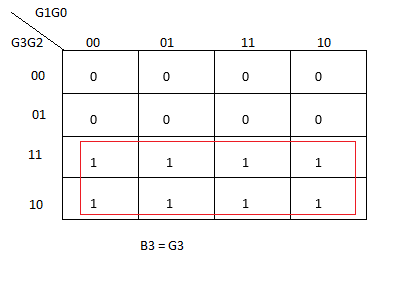As the first preference should be given to eight pair element, the above mapping is done as per 8 pair mapping.

The Gray code equivalent for B3 is G3.

Code Converter | BCD -> Excess-3 Converter

K-map for B2: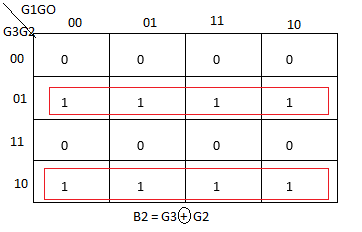The second preference should be given to 4 paired elements if there are no 8 paired elements. As this rule, the above k – mapping is done for 4 pair elements.

The red framed boxes denote the Mapping of elements.

Code Conversion | Binary -> Gray Code Converter

K-map for B1: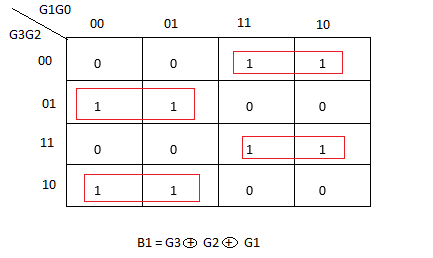Next, to 4 pair elements, the preference should be given to 2 pair elements. As per this rule, the k -mapping is done for B1.

Logic Gates | Logic Gate Symbols | diagram | Truth Table

K-map for B0: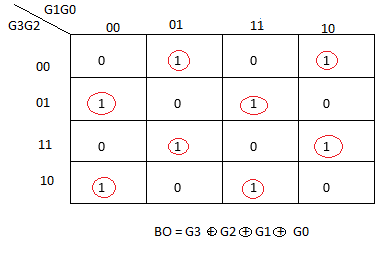Next, to 2 pair elements, the preference should be given to 1 element. As per this rule, the k – mapping is done for B0.

## Logic Diagram: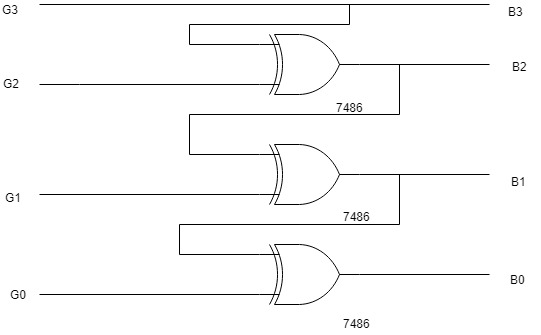The following logic diagram is for Gray code to binary converter.

(In the above conversion, there is no necessity for don’t care conditions, but in other conversions like BCD to Excess-3 there is a need for don’t care conditions in order to proceed k-mapping.)

The Different logic diagram can be drawn by using Combination based on simplified outputs.

You may also like:

Process | wind power plant?

Layout | Thermal Power Plant?

Layout | Nuclear Power Plant?

Layout | Hydro-electric Power Plant?

Methods of Neutral Grounding | Neutral Earthing